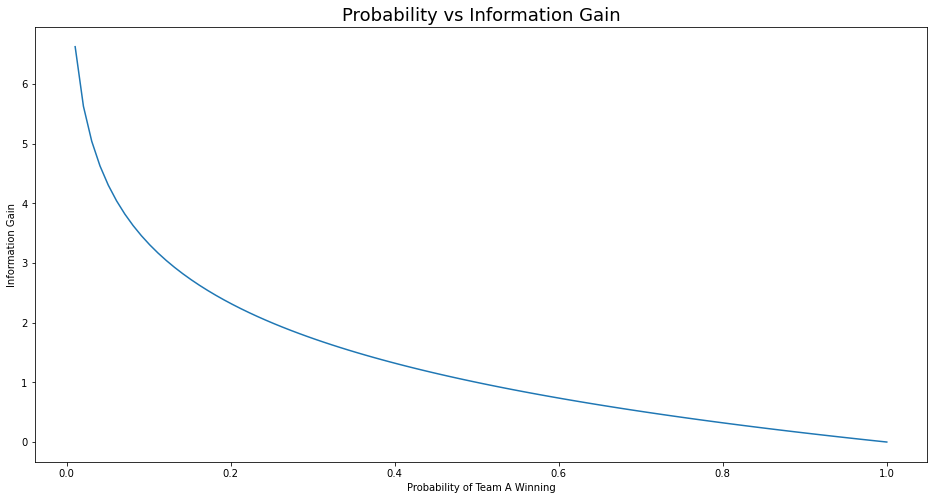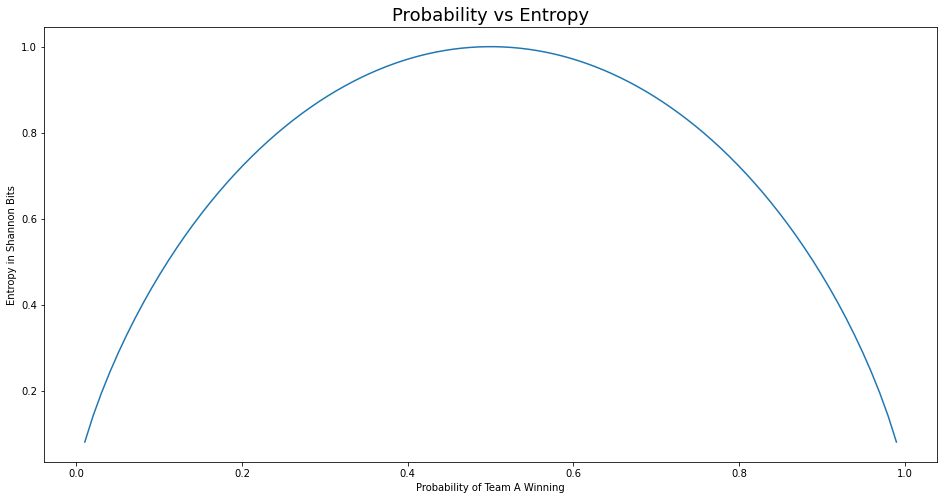### The Best Way to Minimize Uncertainty is NOT Being Informed, Surprised? Let us Measure Surprise

Posted January 14, 2022 by Gowri Shankar  ‐  6 min read

Ignorance is bliss. We all know there is a deeper meaning to this phrase from a philosophical context that points towards lethargic attitude. I would like to define the word ignorance as a lack of knowledge or information. Often we believe the more information we have, the more we are certain about the past, present, and future events associated with that information. Information theory differs significantly on that belief, Thanks to Claude Shannon. i.e. the more the information we have, the more we fill the uncertainty bucket that we detest. Is there any fun in knowing that an event is absolutely certain to happen? for example, Proteas won the series(Cricket) against India. The improbable state of events brings more information which is the cause for all surprises to keep us sitting on the edge of the seat. Test cricket - Game of glorious uncertainties after all..! Hence, we shall learn more about surprises especially measuring surprises.

We have discussed entropy, the measure of uncertainty in the past via more than 5 different posts. This post is a short one that is going to describe Surprise in large. Ideally, all the posts under the topic should be renamed to Information Theory but I am afraid it will break the references. Hence I am starting a new sub-section called Information Theory under Probabilistic ML/DNN. Previous posts can be referred to here,

### Objective

The main objective of this post is to understand What is Entropy and How it is related to Probability? in the context of information theory.

## Introduction

The first and foremost thing in information theory is the fact that information is uncertain. More the information, more the uncertainty - Information leads to an increase or decrease in uncertainty. Then entropy is the measure of uncertainty. Claude Shannon in his book titled A mathematical theory of communication(1948) coined entropy can be interpreted as the expected information conveyed by transmitting a symbol with a certain probability of that symbol picked from a pool of symbols. Here we can simply interpret an ASCII code as a symbol picked from a set of 256 symbols.

To get a better understanding, if no information is conveyed for an event and the probability of that event to occur is 1 - then its entropy is 0. For example, it is certain that a political leader is destined to win the election then the entropy is zero with no uncertainties. Shannon measured uncertainty using the term bit, we call it Shannon Bit. It is similar to the bit in digital computing represented with either 0 or 1 but never the same at the same time.

Let us take the example of a test match cricket and analyze. When the cricket match is over, information gain is 1 because the outcome is declared(victory or defeat). Until then the a-priori probability of winning(or losing) the match is possible and the possibility of winning(or losing) is higher than losing(or winning) - information conveyed is less than 1. In totality, the information conveyed is heading towards 1 while the event is in progress and this information can never go negative.

Let us rewind a bit, to the moment when the coin was tossed. That is when the uncertainty is maximum because the probability of winning and losing is equal. Shall I say it is the moment of uniform distribution? Yes, a uniform distribution maximizes the uncertainty. i.e $$\Large log_2(N) \tag{1. The entropy of Equiprobable Events}$$ Where,

• $N$ is the number of probable events.

Here you go, the Shannons formulae for uncertainty,

$$\Large H(X) = - \sum_{i=1}^n p_i log_2 p_i \tag{2. Entropy, Measure of Uncertainty in bits/symbol}$$

### Surprise

When we are absolutely certain that the event will occur and then it did not - Is that not a surprise? The idea of surprise and the likelihood of an event are closely related. On reflection, the surprise is inversely proportional to probability. $$\Large Surprise \propto \frac {1}{Probability} \tag{3. Surprise vs Probability}$$

Simply speaking, if the probability is low there exists a significantly large amount of surprise. Further, the awareness of the confounders of an unlikely event is more informative than the most likely event. This beats the common beliefs and assumptions of human beings when it comes to information.

Is it wise to conclude more the information, more the anxiety? I will just leave it to the reader’s discretion saying just chill.

Let us consider 3 situations in a test cricket match between Team A and Team B where $P_A$ is the probability of Team A winning the match,

• When the coin was tossed, $(P_A = 0.5)$
• End of the first innings with Team A having a lead of 15 runs $(P_A = 0.55)$
• End of the first innings with Team A having a lead of 150 runs $(P_A = 0.9)$
• End of the match when Team A’s victory is declared $(P_A = 1)$

From the above data, we can see conclude the accuracy of predicting Team A’s victory is ${50%, 55%, 90%, 100% }$. Further, we shall calculate the information gain as follows $$Information \ Gain = log_2(1/P_A) \tag{4. Information Gain in Bits}$$

import numpy as np
p_A = np.array([0.5, 0.55, 0.9, 1])
information_gain = np.log2(1/p_A)
information_gain

array([1.        , 0.86249648, 0.15200309, 0.        ])


The above scenario of a test match is considered to be a fair game, hence at the time of the toss, we have the maximum information gain of 1 Shannon bit and fall to 0 when the result is declared.

Let us examine an unfair game where the probability is not in favor of Team A, considering this analysis is conducted before the coin toss. Information gain is more than 6 when the probability of Team A’s victory is zero. This proves more the information is more the uncertainty of the outcome.

import matplotlib.pyplot as plt
plt.figure(figsize=(16, 8))
probabilities = np.linspace(0, 1, num=100)
information_gain = np.log2(1/probabilities)
plt.plot(probabilities, information_gain)
plt.xlabel("Probability of Team A Winning")
plt.ylabel("Information Gain")
plt.title("Probability vs Information Gain", fontsize=18)
plt.show()

/var/folders/wh/h43cl57j4ljf1x5_4p1bqmx80000gn/T/ipykernel_4156/3910785934.py:4: RuntimeWarning: divide by zero encountered in true_divide
information_gain = np.log2(1/probabilities)### Observations From a Test Match

From $eqn.2$ let us observe what happens to entropy during various stages(in terms of probability of Team A winning) of the match. $$\Large H = -P_A * log_2(p_A) - (1 - P_A) * log(1 - P_A) \tag{5. Entropy of a binary outcome event}$$

We shall calculate entropy using a lambda function and observe how it is related to probability.

f = lambda p: -p * np.log2(p) - (1-p)*np.log2(1-p)
entropy = f(probabilities)
plt.figure(figsize=(16, 8))
plt.plot(probabilities, entropy)
plt.xlabel("Probability of Team A Winning")
plt.ylabel("Entropy in Shannon Bits")
plt.title("Probability vs Entropy", fontsize=18)
plt.show()

/var/folders/wh/h43cl57j4ljf1x5_4p1bqmx80000gn/T/ipykernel_4156/656302744.py:1: RuntimeWarning: divide by zero encountered in log2
f = lambda p: -p * np.log2(p) - (1-p)*np.log2(1-p)
/var/folders/wh/h43cl57j4ljf1x5_4p1bqmx80000gn/T/ipykernel_4156/656302744.py:1: RuntimeWarning: invalid value encountered in multiply
f = lambda p: -p * np.log2(p) - (1-p)*np.log2(1-p)## Epilogue

Though the title of this post is pompous about surprise and measuring surprise, the truth is entropy and surprise are the same. The notion of entropy first appeared in thermodynamics during the mid of $18^{th}$ century(Carnot Engine). Then Claude Shannon took it to its glory in the context of Information Theory. The key takeaway from this post is that information can be measured like any other commodity(eg, mass, energy, etc) and the information is maximum when the possibility of events occurring is uniformly distributed.